Like   Tweet   Pin   +1   in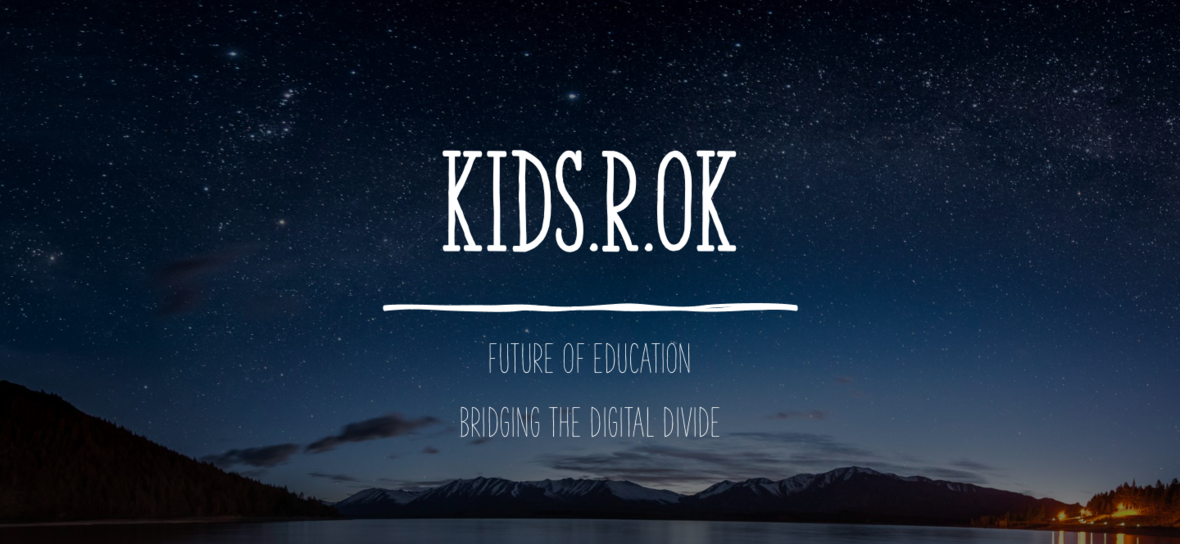table div table+table div table{width:100%;padding:0}table div table+table div table img{width:96.23%;padding:0;float:none}table div table+table div table td{width:100%;padding:0 1.88% 18px}/* styles *//* styles */ Dear Friend of kids.R.ok Hope you are all well and safe during this unprecedented year, which probably will go down in history as a turning point. What actions we take now will determine the course of tomorrow and the lessons we can learn from today will create a better future. We have focused on bridging the digital divide with online classes and initiatives that benefit the children in the lower strata of the economy. Kids.R.ok would like to specially thank our team in India, led by our Project Manager, Evangelin Grace and coordinated by our teachers under the leadership of our Indian Director Sunjeet Sudhir, to keep all our projects on track and keep the safety protocol on the ground in spite of many obstacles. The positive attitude of our team has indeed trickled down to the children who living under months of lockdown in their homes and their parents facing economic difficulty only added to their burden of survival. One of our children's father unfortunately passed away due to covid-19.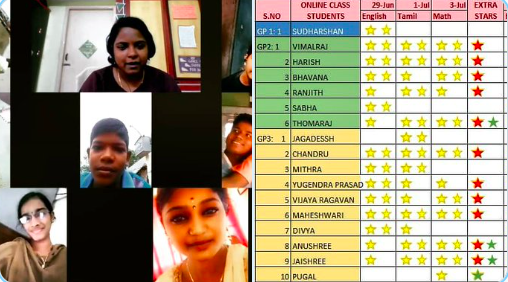As we encourage more children to participate in the online class, we use a online scoring metric giving stars on the board for children who complete their assignments dutifully

From closing down our onsite education centers in early March for safety reason to now seeing thriving online programs where children are able to learn new things through Zoom and Whatsapp platforms. The online programs has been very rewarding because time is not lost for children to continue to learn and their parents said how thankful they were to see their kids learning at home.

 /* styles */ A positive story we want to share from one of our projects. A young boy named Vignesh was struggling to perform well in school and didn't want to continue studying high school after his 10th grade which he barely managed to pass on multiple attempts. His mother came to us crying and asked us to intervene. When we enquired with him, the reason for his decision was that he saw his friends dropout from school get labor jobs and already started making money. We did a lot of counselling with him and his parents to make him see the long term view of investing his time in educating himself further. Thankfully, he listened and continued to pursue his education with a lot of hard work and extra support from our teachers. Today we are happy to announce he has graduated from high school this year and got admitted at a University to study further. This is our motto, no child left behind.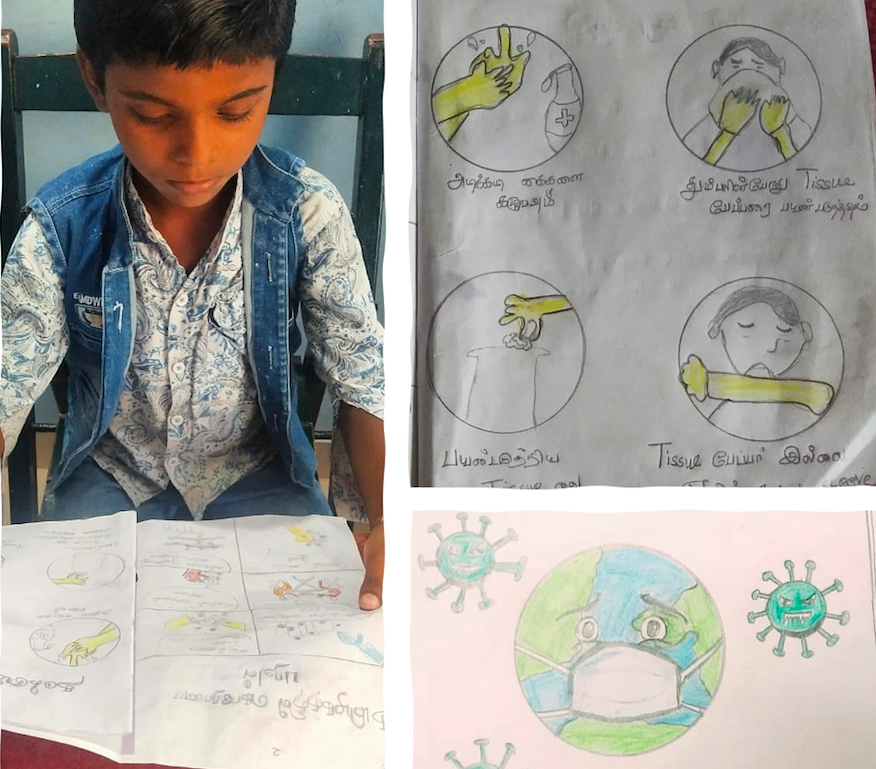We would like to thank our well wishers and donors for your continued support this year. We managed to feed 110 daily wage earning families living in the slums where our children come from for two full months. We deployed a four phases intervention being: Psycho Social support, Online education support, Staff development support and Economical support. We ran many online career guidance and counselling sessions for both children and parents, sending extra resources for our teachers to help bring children back on track to learn.

 /* styles */ Reminding everyone again that we can overcome all types of challenges when we work together, remain strong, encouraging everyone and showing love in times of darkness. With much gratitude, kids.R.ok Team
 table div table+table+table+table+table+table+table+table+table div table{width:100%;padding:0}table div table+table+table+table+table+table+table+table+table div table img{width:96.23%;padding:0;float:none}table div table+table+table+table+table+table+table+table+table div table td{width:100%;padding:0 1.88% 18px}/* styles */## COVID-19 Continued Response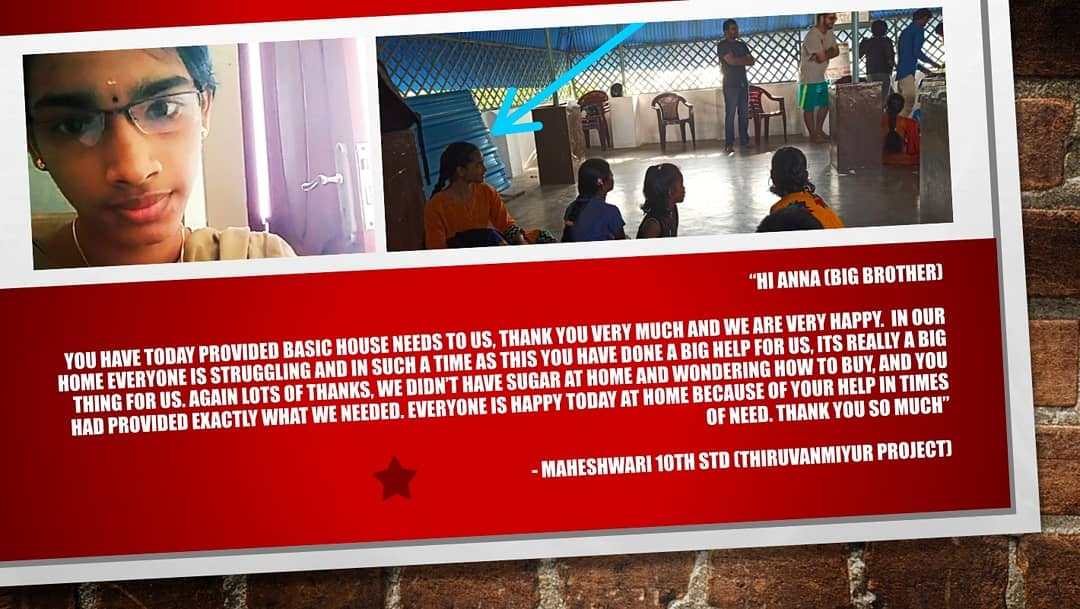"I’m very grateful to all our friends and donors who have really come out in such a generous fashion and provided such support to all our children’s families" Sunjeet Sudhir - Kids.R.ok Co-Founder and Director India
 table div table+table+table+table+table+table+table+table+table+table+table+table div table{width:100%;padding:0}table div table+table+table+table+table+table+table+table+table+table+table+table div table img{width:96.23%;padding:0;float:none}table div table+table+table+table+table+table+table+table+table+table+table+table div table td{width:100%;padding:0 1.88% 18px}/* styles */## Change Makers

 table div table+table+table+table+table+table+table+table+table+table+table+table+table+table div table,table.module-13{width:47.36%;float:left;padding:0}table div table+table+table+table+table+table+table+table+table+table+table+table+table+table div table a{border:0 none;text-decoration:none}table div table+table+table+table+table+table+table+table+table+table+table+table+table+table div table img{width:100%!important;border:0 none;text-decoration:none}table div table+table+table+table+table+table+table+table+table+table+table+table+table+table div table td{width:100%;padding:0 20px 20px 0}/* styles */ Change-makers!! Esther is our teacher at Saidapet center and her dream is to create good leaders and sees children as our future. It's a privilege to have people like her on kids.R.ok team. Read more about her story here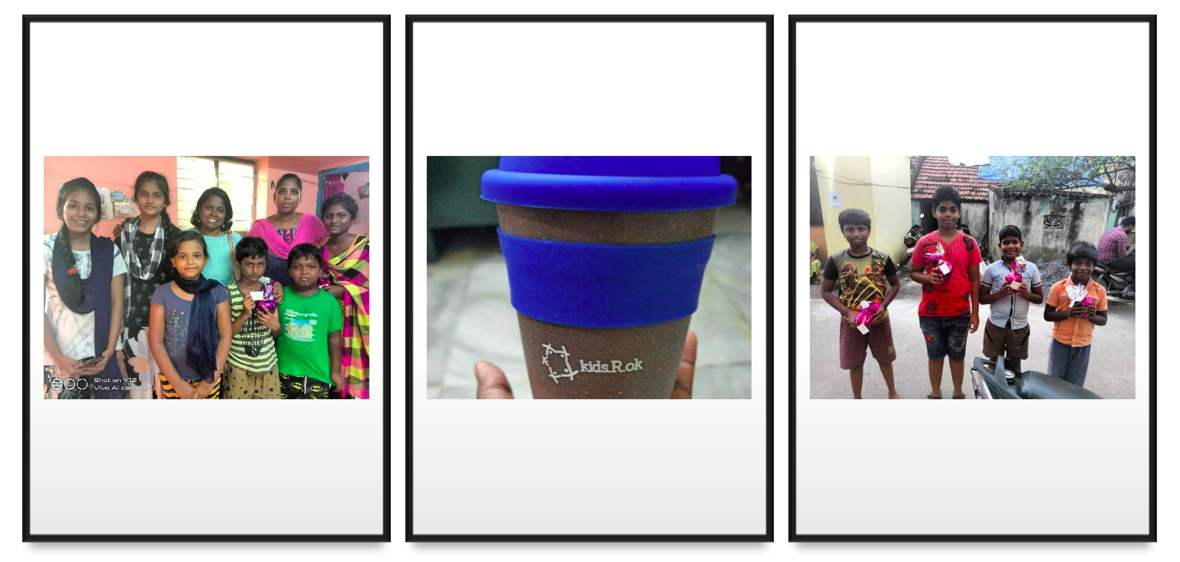A little something to keep the spirits up
 /* styles */ After a marathon virtual talent show, where the kids in their homes with great enthusiasm showed various skills and talents. We reached out to meet them in person in their homes and give a small gift.
 table div table+table+table+table+table+table+table+table+table+table+table+table+table+table+table+table+table div table{width:100%;padding:0}table div table+table+table+table+table+table+table+table+table+table+table+table+table+table+table+table+table div table img{width:96.23%;padding:0;float:none}table div table+table+table+table+table+table+table+table+table+table+table+table+table+table+table+table+table div table td{width:100%;padding:0 1.88% 18px}/* styles */## MENTORSHIP PROGRAM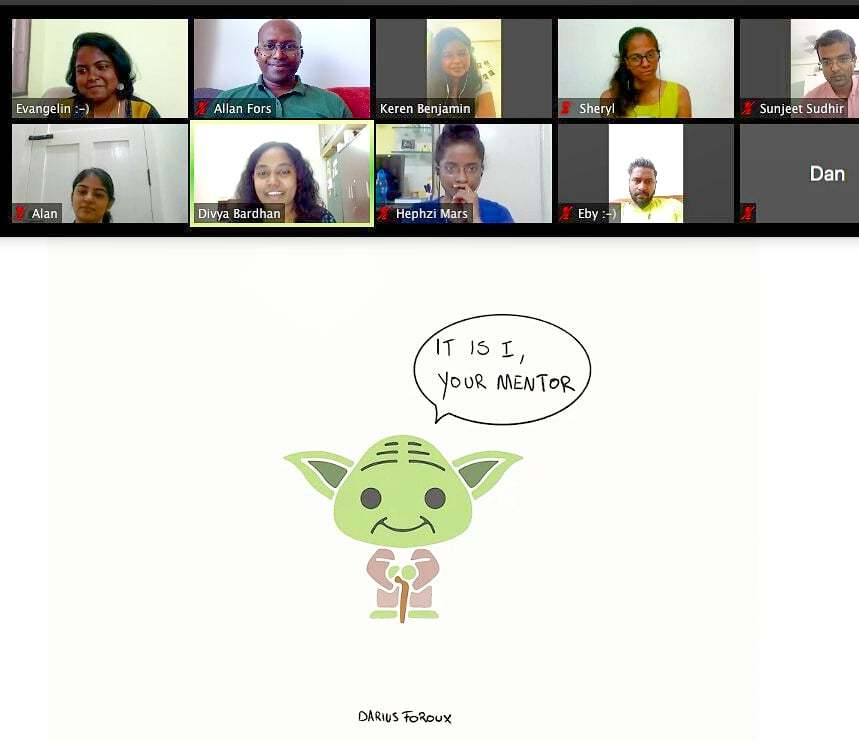/* styles */ Kids.R.ok just kick started our 'Mentorship Program' with a cohort of 16 volunteers. We got to know the mentors and linked them up with a respective mentee from each of our centers. This program is for our children who are between ages 14-18. We are grateful to have such passionate, empathetic and inspiring people to lead the selected kids during the 8 weeks mentoring program.
 table div table+table+table+table+table+table+table+table+table+table+table+table+table+table+table+table+table+table+table+table+table div table{width:100%;padding:0}table div table+table+table+table+table+table+table+table+table+table+table+table+table+table+table+table+table+table+table+table+table div table img{width:96.23%;padding:0;float:none}table div table+table+table+table+table+table+table+table+table+table+table+table+table+table+table+table+table+table+table+table+table div table td{width:100%;padding:0 1.88% 18px}/* styles */## kids.R.ok Activities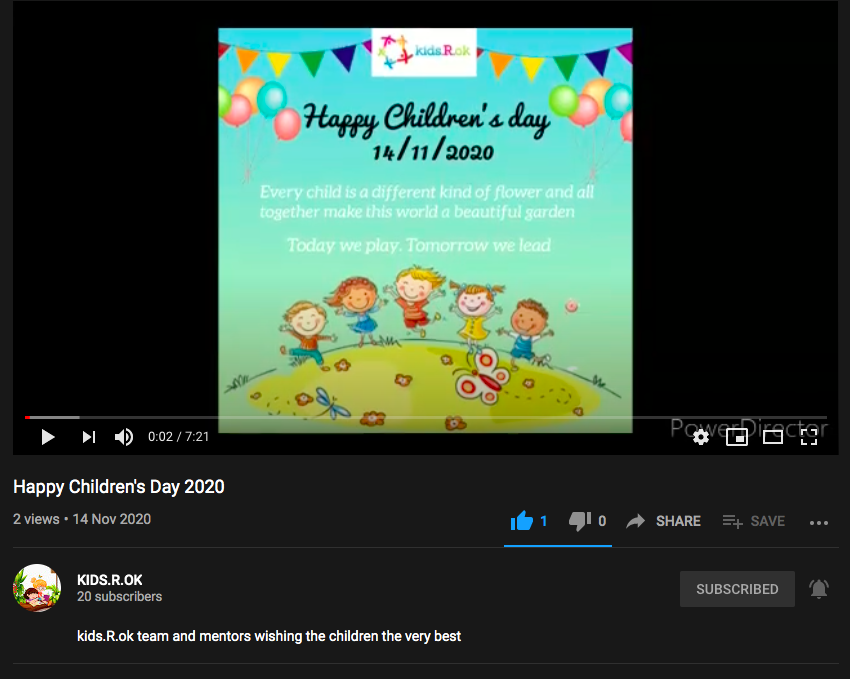YOUTUBE VIDEO - CHECKOUT THE WISHES OF OUR TEAM TO THE CHILDREN
 table div table+table+table+table+table+table+table+table+table+table+table+table+table+table+table+table+table+table+table+table+table+table+table+table div table{width:100%;padding:0}table div table+table+table+table+table+table+table+table+table+table+table+table+table+table+table+table+table+table+table+table+table+table+table+table div table img{width:96.23%;padding:0;float:none}table div table+table+table+table+table+table+table+table+table+table+table+table+table+table+table+table+table+table+table+table+table+table+table+table div table td{width:100%;padding:0 1.88% 18px}/* styles */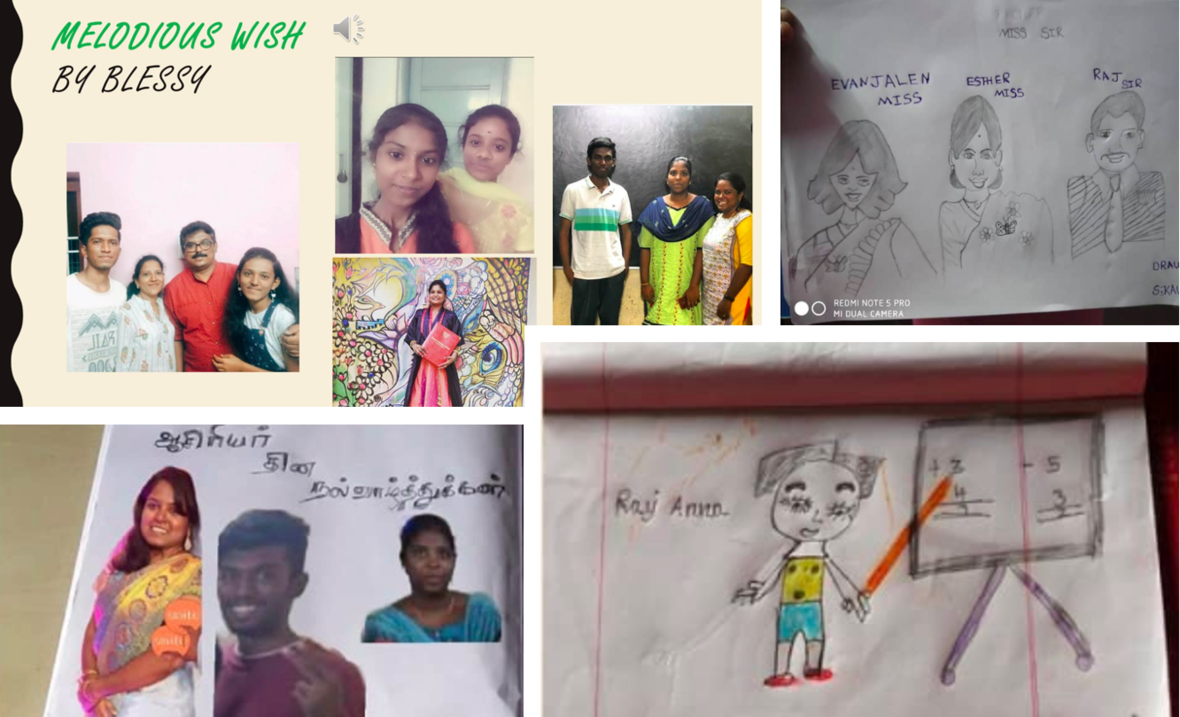Artwork done by our children on Teachers Appreciation Day
 table div table+table+table+table+table+table+table+table+table+table+table+table+table+table+table+table+table+table+table+table+table+table+table+table+table+table div table{width:100%;padding:0}table div table+table+table+table+table+table+table+table+table+table+table+table+table+table+table+table+table+table+table+table+table+table+table+table+table+table div table img{width:96.23%;padding:0;float:none}table div table+table+table+table+table+table+table+table+table+table+table+table+table+table+table+table+table+table+table+table+table+table+table+table+table+table div table td{width:100%;padding:0 1.88% 18px}/* styles */## Learning by doing

 table.module-27{width:99.81%;padding:0}table div table+table+table+table+table+table+table+table+table+table+table+table+table+table+table+table+table+table+table+table+table+table+table+table+table+table+table+table div table{width:99.81%;float:none;margin-left:auto;margin-right:auto;padding:0}table div table+table+table+table+table+table+table+table+table+table+table+table+table+table+table+table+table+table+table+table+table+table+table+table+table+table+table+table div table a{border:0 none;text-decoration:none}table div table+table+table+table+table+table+table+table+table+table+table+table+table+table+table+table+table+table+table+table+table+table+table+table+table+table+table+table div table img{width:100%!important;border:0 none;text-decoration:none}table div table+table+table+table+table+table+table+table+table+table+table+table+table+table+table+table+table+table+table+table+table+table+table+table+table+table+table+table div table td{width:100%;padding:0}/* styles */
 /* styles */ Look at some fine artwork by the kids and few videos they sent based on our online extra circular teaching activity ✌

## Math & Science with interactive learning:

Exploring and learning about space! We are using interactive educational tools to make science and math easy & fun to learn.

 table div table+table+table+table+table+table+table+table+table+table+table+table+table+table+table+table+table+table+table+table+table+table+table+table+table+table+table+table+table+table+table div table td,table.module-30{width:100%;padding:0}table div table+table+table+table+table+table+table+table+table+table+table+table+table+table+table+table+table+table+table+table+table+table+table+table+table+table+table+table+table+table+table div table{width:100%;float:none;margin-left:auto;margin-right:auto;padding:0}table div table+table+table+table+table+table+table+table+table+table+table+table+table+table+table+table+table+table+table+table+table+table+table+table+table+table+table+table+table+table+table div table a{border:0 none;text-decoration:none}table div table+table+table+table+table+table+table+table+table+table+table+table+table+table+table+table+table+table+table+table+table+table+table+table+table+table+table+table+table+table+table div table img{width:100%!important;border:0 none;text-decoration:none}/* styles */
 table div table+table+table+table+table+table+table+table+table+table+table+table+table+table+table+table+table+table+table+table+table+table+table+table+table+table+table+table+table+table+table+table div table{width:100%;padding:0}table div table+table+table+table+table+table+table+table+table+table+table+table+table+table+table+table+table+table+table+table+table+table+table+table+table+table+table+table+table+table+table+table div table img{width:96.23%;padding:0;float:none}table div table+table+table+table+table+table+table+table+table+table+table+table+table+table+table+table+table+table+table+table+table+table+table+table+table+table+table+table+table+table+table+table div table td{width:100%;padding:0 1.88% 18px}/* styles */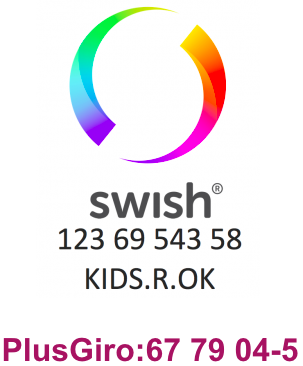Thank you donors and friends of KIDS.R.OK for your support and encouragement to bring the dreams of these children growing up in the underprivileged parts of India come true

Kids.R.ok Team

 table div table+table+table+table+table+table+table+table+table+table+table+table+table+table+table+table+table+table+table+table+table+table+table+table+table+table+table+table+table+table+table+table+table+table+table div table{width:100%;padding:0}table div table+table+table+table+table+table+table+table+table+table+table+table+table+table+table+table+table+table+table+table+table+table+table+table+table+table+table+table+table+table+table+table+table+table+table div table img{width:96.23%;padding:0;float:none}table div table+table+table+table+table+table+table+table+table+table+table+table+table+table+table+table+table+table+table+table+table+table+table+table+table+table+table+table+table+table+table+table+table+table+table div table td{width:100%;padding:0 1.88% 18px}/* styles */